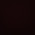## Monday, April 14, 2014

### LCM Type 2 Question Solving - Video Lecture

The generalisation of Least Common Multiple Type 2 is as follows -

What would be the form of a number N which when divided by a,b,c will leave a remainder of p,q,r respectively ?

So the generalisation would be of this form -

{ LCM (a,b,c) }k  +  Remainder

where k is a constant. The function of this k is to calculate the value of this expression according to the condition provided in the question. Basically in order to find out the least possible number of the form N, we will simply substitute k with 1 and for higher values we will proceed accordingly.

Question - A number N when divided by 3,4,5 leaves a remainder of 2,3,4 respectively.

1. What is the least possible number of the form N ?

2. What is the least possible 4 digit number of the form N ?

3. What is the largest possible 4 digit number of the form N ?

1.thank u sir........

2.thank u sir.......

3.thank you sir....you are really great.

4.5.Sir your videos are making my concept more clear and understandable. Thank you so much, Sir.